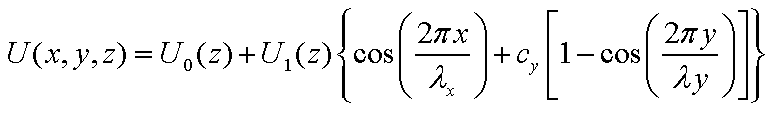# Help with surface potential

Dear all,

I’m modeling the adsorption of paraffin molecules onto a surface. I would like to apply the following surface potential to one boundary of my cubic simulation boxbut I’m not sure how to do this. The potential is a function of x and y as well as z(the distance from the surface). Any help will be greatly appreciated and thank you all in advance.

If you mean this is a potential that will induce force

on atoms, then you can look at the fix addforce command.

It lets you define per-atom variables for energy and force

that are applied to each atom, based on plugging its x,y,z

coords into equations like the above.

If that is too complicated or too slow for your application,

then you can code it yourself as a “fix”, and add it to LAMMPS.

SteveIf you mean this is a potential that will induce force
on atoms, then you can look at the fix addforce command.
It lets you define per-atom variables for energy and force
that are applied to each atom, based on plugging its x,y,z
coords into equations like the above.

If that is too complicated or too slow for your application,
then you can code it yourself as a "fix", and add it to LAMMPS.

Hi Mark.
I just wrote this email to someone else trying to implement an
idealized slab, so I might as well forward it to you as well. There
might be some mistakes in this post, but hopefully I don't lead you
astray.

Suppose that you want to adds a force to each charged particle
based on its distance to a surface. IF you have ALREADY tried using
fix addforce, and decided that it is not suitable, then you could

existing fixes, such as "fix_addforce.cpp" or "fix_wall.cpp".

Suppose we want to call our new fix "gouychapman"
(Gouy-Chapman is an old continuum model used to calculate the
interaction of a charged particle near a charged surface in solvent)
Anyway, to do that:
cd to the "src/" directory:

You can do this using a text-editor, or using "sed"

The main modification you would need to make is to
replace the following lines with the mathematical formula
for the force acting on each atom. The relevant lines in

xvalue = input->variable->compute_equal(xvar);
yvalue = input->variable->compute_equal(yvar);
zvalue = input->variable->compute_equal(zvar);
f[i]=xvalue;
f[i]=yvalue;
f[i]=zvalue;

...Your new code should replace this with 3 formulas
involving these quantities:
atom->x[i], atom->x[i], atom->x[i],
and, generally speaking, the charge, atom->q[i]

You will have to delete a lot of code specific to fix_addforce.cpp
which you don't need. For those details see below.
For more details, consult the documentation here.
http://lammps.sandia.gov/doc/Developer.pdf

...and look at other fix examples (eg "fix_wall.cpp").

Cheers
Andrew:

---- more details ----
If you are starting from fix_addforce.cpp, then you would
probably want to clear out all of the stuff which specifies
the various kinds of LAMMPS-variables used in the mathematical
expression ("varflag", "xstyle", "ystyle" and "zstyle"):
As such, my guess is that these lines are probably not relevant:
input->variable->compute_atom(xvar,igroup,&sforce,4,0);
input->variable->compute_atom(yvar,igroup,&sforce,4,0);
input->variable->compute_atom(zvar,igroup,&sforce,4,0);
input->variable->compute_atom(evar,igroup,&sforce,4,0);

The new constructor would probably look something which vaguely
resembles this:

FixGouyChapman::FixGouyChapman(LAMMPS *lmp, int narg, char **arg) :
Fix(lmp, narg, arg)
{
nevery=1; # so it gets executed every time step
force_flag = 0;
foriginal = foriginal = foriginal = foriginal = 0.0;
maxatom = atom->nmax;
}

This does not get rid of all of the junk that was in fix_addforce.cpp,
but it's a start. Hopefully this points you in the right direction.

Again, you're probably better off using fix_wall.cpp as a starting
template, because it is a little bit simpler.

Cheers again